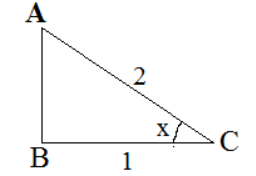QUESTION

# Find the value of $\sin x,tanx,\cot x$ and $\cot x+\csc x$, If $\sec x=2$

Hint: Draw a figure where ABC be a right angled triangle where $\angle B=90{}^\circ$ and $\angle C=x{}^\circ$. Now, use $\sec \theta =\dfrac{\text{Hypotenuse}}{\text{Adjacent side}}$ and the Pythagoras theorem to find AB. Next use various trigonometric formulae: $\sin \theta =\dfrac{\text{Opposite side}}{\text{Hypotenuse}}$, $\tan \theta =\dfrac{\text{Opposite side}}{\text{Adjacent side}}$, $\cot x=\dfrac{1}{\tan x}$ and $\csc x=\dfrac{1}{sinx}$ to find the final answer.

Complete Step-by-Step solution:
In this question, we are given that $\sec x=2$.
Using this information, we need to find the value of $\sin x,tanx,\cot x$ and $\cot x+\csc x$
Let ABC be a right angled triangle where $\angle B=90{}^\circ$ and $\angle C=x{}^\circ$ as shown in the figure:Now it is given that $\sec x=2$ and we know that, in a right angled triangle, $\sec \theta$ is equal to hypotenuse over adjacent side, i.e. $\sec \theta =\dfrac{\text{Hypotenuse}}{\text{Adjacent side}}$ ​, therefore, hypotenuse AC = 2 and adjacent side BC = 1.
Now, we will use Pythagoras theorem in triangle ABC.
In mathematics, the Pythagorean theorem, also known as Pythagoras' theorem, is a fundamental relation in Euclidean geometry among the three sides of a right triangle. It states that the square on the hypotenuse of a right angled triangle is equal to the sum of the squares of the other two sides.
i.e. ${{a}^{2}}+{{b}^{2}}={{c}^{2}}$, where c is the hypotenuse and a and b are the other sides.
We have the following:
$A{{C}^{2}}=A{{B}^{2}}+B{{C}^{2}}$
${{2}^{2}}=A{{B}^{2}}+{{1}^{2}}$
$A{{B}^{2}}=3$
$AB=\sqrt{3}$
Therefore, the opposite side, $AB=\sqrt{3}$
We know that, in a right angled triangle,
$\sin \theta$ is equal to opposite side divided by hypotenuse, i.e. $\sin \theta =\dfrac{\text{Opposite side}}{\text{Hypotenuse}}$ ​ and,
$\tan \theta$ is equal to opposite side divided by adjacent side, i.e. $\tan \theta =\dfrac{\text{Opposite side}}{\text{Adjacent side}}$
Using these formulae, we have the following:
$\sin x=\dfrac{\text{Opposite side}}{\text{Hypotenuse}}=\dfrac{AB}{AC}=\dfrac{\sqrt{3}}{2}$
$\tan x=\dfrac{\text{Opposite side}}{\text{Adjacent side}}=\dfrac{AB}{BC}=\dfrac{\sqrt{3}}{1}=\sqrt{3}$
$\cot x=\dfrac{1}{\tan x}=\dfrac{1}{\sqrt{3}}$
$\csc x=\dfrac{1}{sinx}=\dfrac{1}{\tfrac{\sqrt{3}}{2}}=\dfrac{2}{\sqrt{3}}$
$\cot x+\csc x=\dfrac{1}{\sqrt{3}}+\dfrac{2}{\sqrt{3}}=\dfrac{3}{\sqrt{3}}=\sqrt{3}$
Hence, the final answer is $\sin x=\dfrac{\sqrt{3}}{2},\tan x=\sqrt{3},\cot x=\dfrac{1}{\sqrt{3}},\left( \cot x+\csc x \right)=\sqrt{3}$

Note: In this question, it is very important to draw the figure to understand the things better. It is very important to know the various trigonometric relations and formulae. Without these, it would be very difficult to solve the question. It is also important to know Pythagoras theorem to find the length of AB.